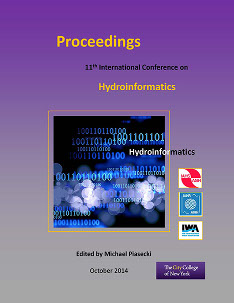## International Conference on Hydroinformatics

Presentation

8-1-2014

#### Abstract

Uncertainty analysis is useful to define the level of reliability of a modelling application, but operational methods are needed to identify the best modelling structure for a specific problem based on uncertainty reduction criteria. One interesting example is given by flood damage estimation problem where different possible modelling solution and flood damage estimation can be used depending on the case study. Past literature showed that several modelling structures may be equally reliable in terms of calibration ability but they may produce different uncertainty levels. The use of the Bayesian approach for uncertainty analysis has been stimulated by its rigorous theoretical framework and by the possibility of evaluating the impact of new knowledge on the modelling predictions. Model structure uncertainty is associated with the assumptions reflected in model conceptualization and mathematical structure. An unfortunate truth in model development is that no matter how many resources are invested in developing a particular model, there remain conditions and situations in which the model is unsuitable to give an accurate forecast. Reliance on a single model typically overestimates the confidence and increases the statistical bias of the forecast. Bayesian model-averaging (BMA) techniques look to overcome the limitations of a single model by linearly combining a number of competing models into a single new model forecast. This method showed that a pooled forecast of competing models outperformed any single model forecast. The early applications of model-averaging for hydrological systems resulted in a point forecast. An extension of these approaches uses a multiple linear regression model to compute model weights while assuming a model Gaussian distribution, which allows for a probabilistic performance evaluation. In the present paper, BMA was applied to several flood damage estimation models in order to identify the best combination of models to analyse urban flooding distribution in Palermo city centre (Italy).